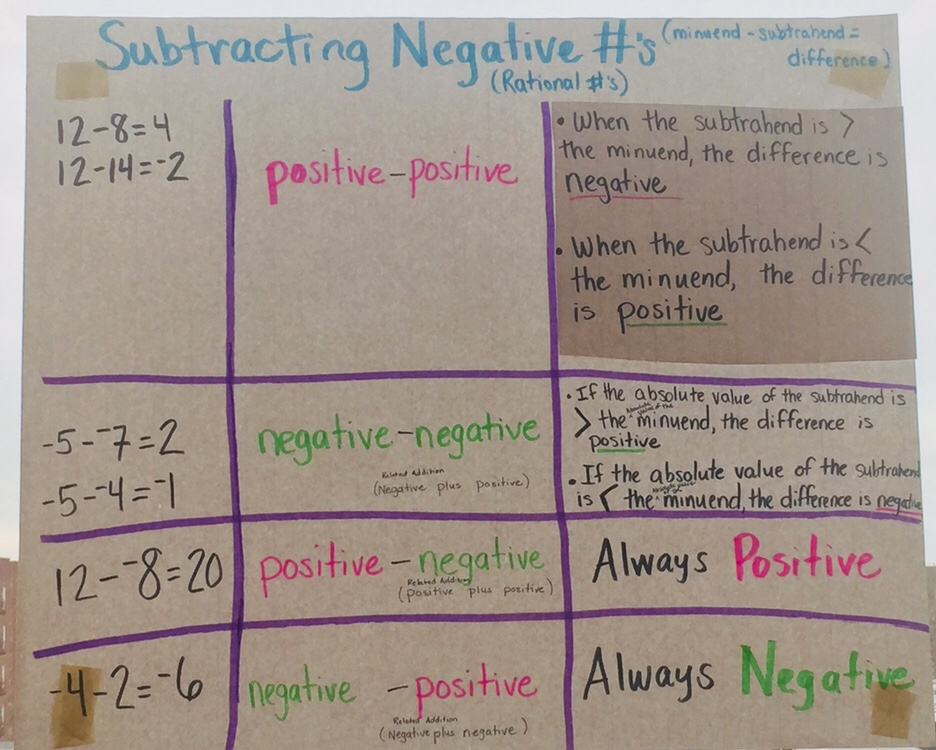# Grade 6 Math week of 1/16/18

In the last week, we’ve been investigating how to add and subtract rational numbers*.  We looked for patterns and began to realize why the results are positive or negative and understand how to calculate the equations.

To do this we used number lines and ‘chip boards’ as tools. The chip boards were physical representations of the positive and negative numbers representing negative quantities as red chips, and positive quantities as black chips. For some students who did not already have an understanding of how addition and subtraction of negative numbers worked, these tools helped students to understand not just how to calculate an equation such as (-14) – (-17) but why the result is a positive 3.

The images below are our notes related to the addition and subtraction combinations that exist, and how to understand what you are looking for in calculation of these situations.This week we will investigate how multiplication and division work when rational numbers are included in these operations and compile our observations.